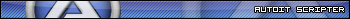# Pong AI's

## Recommended Posts

Hiya!

I was interested in making AI's, so I thought I would start easy.

Tell me what you think of these Pong AI's.

```#include <GUIConstantsEx.au3>

; Normal player movement speed = 5

Global \$Hard_x = 20, \$Hard_Y = 100
Global \$Med_x = 120, \$Med_Y = 300
Global \$Easy_x = 220, \$Easy_Y = 100
Global \$Ball_x = Random(300, 500, 1), \$Ball_y = Random(50, 450, 1)
Global \$Ball_SpeedX = 6, \$Ball_SpeedY = 3
Global \$Direction = False
Global \$HardBlocks = 0, \$MedBlocks = 0, \$EasyBlocks = 0, \$Fails = 0

\$GUI = GUICreate("Pong AI's > | Hard | Medium | Easy", 800, 480)
\$ScoreLabel = GUICtrlCreateLabel("", 702, 422, 120, 50)

\$Hard = GUICtrlCreateGraphic(\$Hard_x, \$Hard_y, 10, 100)
GUICtrlSetGraphic(\$Hard, \$GUI_GR_COLOR, 0xFF0000, 0xFF0000)
GUICtrlSetGraphic(\$Hard, \$GUI_GR_RECT, 0, 0, 10, 100)
GUICtrlSetGraphic(\$Hard, \$GUI_GR_REFRESH)
GUICtrlSetGraphic(\$Hard, \$GUI_GR_CLOSE)

\$Med = GUICtrlCreateGraphic(\$Med_x, \$Med_y, 10, 100)
GUICtrlSetGraphic(\$Med, \$GUI_GR_COLOR, 0xFFFF00, 0xFFFF00)
GUICtrlSetGraphic(\$Med, \$GUI_GR_RECT, 0, 0, 10, 100)
GUICtrlSetGraphic(\$Med, \$GUI_GR_REFRESH)
GUICtrlSetGraphic(\$Med, \$GUI_GR_CLOSE)

\$Easy = GUICtrlCreateGraphic(\$Easy_x, \$Easy_y, 10, 100)
GUICtrlSetGraphic(\$Easy, \$GUI_GR_COLOR, 0x00FF00, 0x00FF00)
GUICtrlSetGraphic(\$Easy, \$GUI_GR_RECT, 0, 0, 10, 100)
GUICtrlSetGraphic(\$Easy, \$GUI_GR_REFRESH)
GUICtrlSetGraphic(\$Easy, \$GUI_GR_CLOSE)

\$Ball = GUICtrlCreateGraphic(\$Ball_x, \$Ball_y, 0, 0)
GUICtrlSetGraphic(\$Ball, \$GUI_GR_COLOR, 0x00FFFF, 0x00FFFF)
GUICtrlSetGraphic(\$Ball, \$GUI_GR_PIE, 0, 0, 5, 0, 360)
GUICtrlSetGraphic(\$Ball, \$GUI_GR_REFRESH)
GUICtrlSetGraphic(\$Ball, \$GUI_GR_CLOSE)

GUISetState()
While 1
Switch GUIGetMsg()
Case -3
Exit
EndSwitch

_Hard()
_Med()
_Easy()
_Ball()

Sleep(20)
GUICtrlSetGraphic(\$Ball, \$GUI_GR_REFRESH)
GUICtrlSetData(\$ScoreLabel, "| Hard blocks: " & \$HardBlocks & " |" & @CRLF & "| Med blocks: " & \$MedBlocks & " |" & @CRLF & "| Easy blocks: " & \$EasyBlocks & " |" & @CRLF & "| Fails:      " & \$Fails & " |")
WEnd

Func _Ball()
\$Ball_x += \$Ball_SpeedX
\$Ball_y += \$Ball_SpeedY
GUICtrlSetPos(\$Ball, \$Ball_x, \$Ball_y)

If (\$Ball_y > 475 Or \$Ball_y < 10) Then \$Ball_SpeedY *= -1

If (\$Ball_x > 790 Or \$Ball_x < 5) Then
\$Ball_SpeedX *= -1
\$Direction = Not \$Direction
\$Ball_SpeedY = Random(-8, 8, 1)
EndIf

If (\$Ball_x < 5) Then \$Fails += 1

If (\$Ball_x < \$Easy_x + 20 And \$Ball_x > \$Easy_x And \$Direction) Then
If (\$Ball_y > \$Easy_y And \$Ball_y < \$Easy_y + 100) Then
\$EasyBlocks += 1
\$Ball_SpeedX *= -1
\$Direction = Not \$Direction
EndIf
EndIf

If (\$Ball_x < \$Med_x + 20 And \$Ball_x > \$Med_x And \$Direction) Then
If (\$Ball_y > \$Med_y And \$Ball_y < \$Med_y + 100) Then
\$MedBlocks += 1
\$Ball_SpeedX *= -1
\$Direction = Not \$Direction
EndIf
EndIf

If (\$Ball_x < \$Hard_x + 20 And \$Ball_x > \$Hard_x And \$Direction) Then
If (\$Ball_y > \$Hard_y And \$Ball_y < \$Hard_y + 100) Then
\$HardBlocks += 1
\$Ball_SpeedX *= -1
\$Direction = Not \$Direction
EndIf
EndIf
EndFunc

Func _Hard()
If (\$Ball_y - 45 < \$Hard_y) Then
If (\$Hard_y > 10) Then \$Hard_y -= 5
EndIf

If (\$Ball_y - 45 > \$Hard_y) Then
If (\$Hard_y < 470 - 100) Then \$Hard_y += 5
EndIf

GUICtrlSetPos(\$Hard, \$Hard_x, \$Hard_y)
EndFunc

Func _Med()
For \$i = 0 To 480
If (\$Ball_y == \$i And \$Ball_y - 45 < \$Med_y) Then
If (\$Med_y > 10) Then \$Med_y -= 3.3 ; 3.5 = max, 3 = default
EndIf

If (\$Ball_y == \$i And \$Ball_y - 45 > \$Med_y) Then
If (\$Med_y < 470 - 100) Then \$Med_y += 3.3 ; 3.5 = max, 3 = default
EndIf
Next

GUICtrlSetPos(\$Med, \$Med_x, \$Med_y)
EndFunc

Func _Easy()
If (\$Ball_y > 0 And \$Ball_y < 240 And \$Ball_y - 45 < \$Easy_y) Then
If (\$Easy_y > 10) Then \$Easy_y -= 3.5 ; 3.5 = max, 3 = default
EndIf

If (\$Ball_y > 241 And \$Ball_y < 480 And \$Ball_y - 45 > \$Easy_y) Then
If (\$Easy_y < 470 - 100) Then \$Easy_y += 3.5 ; 3.5 = max, 3 = default
EndIf

GUICtrlSetPos(\$Easy, \$Easy_x, \$Easy_y)
EndFunc```

AlmarMMinesweeper

A minesweeper game created in autoit, source available.

_Mouse_UDF

An UDF for registering functions to mouse events, made in pure autoit.

2D Hitbox Editor

A 2D hitbox editor for quick creation of 2D sphere and rectangle hitboxes.

##### Share on other sites

Well Donei like that you set it to different difficulty levelsCreator Of Xtreme DevelopersPixel Pattern UDFTray GUI UDFMathssend & recive register scriptMouse Control via Webcam##### Share on other sites

although i think using GDIPlus would be easierCreator Of Xtreme DevelopersPixel Pattern UDFTray GUI UDFMathssend & recive register scriptMouse Control via Webcam##### Share on other sites

Hmm realy? I still didnt use GDI+, so... But I will try :]

Minesweeper

A minesweeper game created in autoit, source available.

_Mouse_UDF

An UDF for registering functions to mouse events, made in pure autoit.

2D Hitbox Editor

A 2D hitbox editor for quick creation of 2D sphere and rectangle hitboxes.

## Create an account

Register a new account

• ### Recently Browsing   0 members

×

• Wiki

• Back

• #### Beta

• Git
• FAQ
×
• Create New...